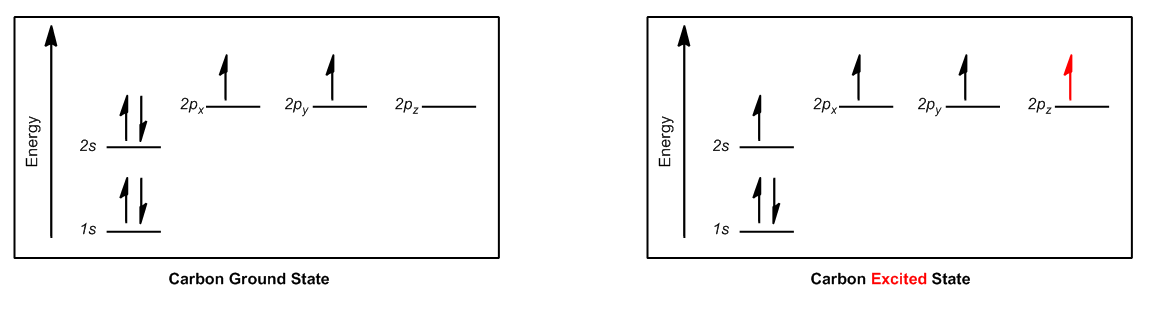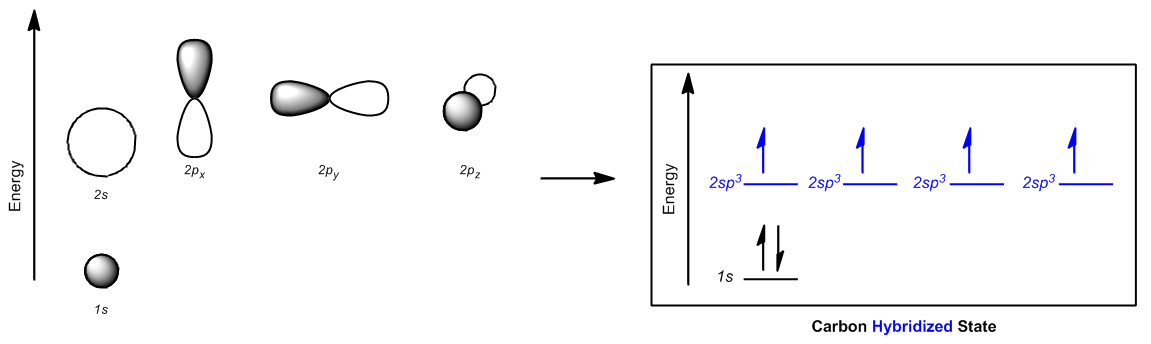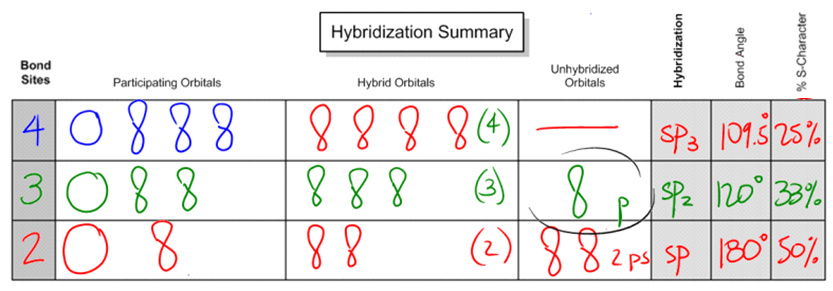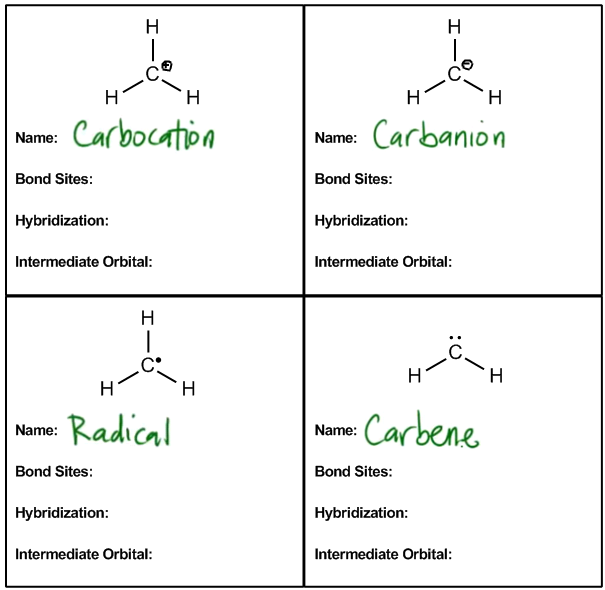Clutch Prep is now a part of Pearson
Ch. 1 - A Review of General ChemistryWorksheetSee all chapters

# Hybridization

See all sections
Sections
Intro to Organic Chemistry
Atomic Structure
Wave Function
Molecular Orbitals
Sigma and Pi Bonds
Octet Rule
Bonding Preferences
Formal Charges
Skeletal Structure
Lewis Structure
Condensed Structural Formula
Degrees of Unsaturation
Constitutional Isomers
Resonance Structures
Hybridization
Molecular Geometry
Electronegativity
Isomer
Polar Vs. Nonpolar
Covalent Bond
Polar Bond

Aufbau Principle predicts that carbon would have two partially filled 2p orbitals, suggesting that carbon could only make 2 bonds. However, carbon actually makes 4 bonds.

How do we explain this?

###### Carbon Excites an Electron

Concept #1: How carbon creates 4 partially-filled orbitals.

Transcript

So the first thing I want to talk about is just the theory of why this even is possible, why this is a big deal.
The Aufbau principle states that electrons can fill orbitals in order of increasing energy. Remember that my orbitals in order of increasing energy were 1s, 2s and then the three 2p's.
If we go ahead and draw the diagram for carbon, let's say. Let's just use carbon as an example. What we're going to find is that the 1s gets completely full at the helium stage. Then the 2s gets completely full at the beryllium stage.
Then, with carbon, we only have two electrons in those p orbitals. Remember that there's three p orbitals total and you only have two electrons in them. By the way, the notation that I'm referring to here has to do with electron configuration, which is something that you guys are supposed to remember from gen chem. So in this case, what that means is that I have two electrons in the 2p orbitals.
So if I were to draw the actual orbital diagrams for both of these what you would realize, or for the carbon, what you would realize is that, like I said, 1s is full, 2s is full, and then the p's are just mess because what I have is basically two unfilled orbitals and then one completely empty orbital.
Remember that I told you guys earlier when we were talking about molecular orbital theory that unfilled orbitals like to bond with other unfilled orbitals in order to make them full and in order to become more stable. So when you're looking at carbon, if it only has two unfilled, like partially filled orbitals, you would think, well why wouldn't it just make two bonds? Why does it make four? Remember this is another way to think about it. We already talked about bonding preferences and stuff, but this actually has to do with orbitals. So why does it make four?
Well, it turns out that this is just a really bad situation for carbon. Carbon does not like to look like this. The reason is because these orbitals are all messed up. The p orbitals, some are partially filled, some are not filled, so that means that it would never really be able to fully stable. So what carbon actually winds up doing is winds up exciting one electron up into one of the p orbitals. So what that does is it actually violates the Aufbau principle and it takes one electron from my 2s orbital and it excites it to one of the 2p orbitals.
So now what I wind up getting is that instead of having one full orbital of lower energy, I'm in three orbitals that kind of just suck. What winds up happening is that I wind up getting four orbitals of higher energy that all are partially filled. Why is that better? That even sounds worse. Now we have an excited electron. That means it has more energy, so why is this better?In terms of stability, now we have fewer filled orbitals, AND we had to excite an electron to do it.  Why is it better to have 4 partially-filled orbitals?

###### Carbon Hybridizes Orbitals

Concept #2: How carbon makes 4 sp3 orbitals.

Transcript

The reason is and this is the crazy thing it turns out that many of these atoms are going to prefer to blend their second shell orbitals together and what that's going to do is it's going to make new hybrid orbitals, OK? So what that means is that instead of the 2S orbital being lower energy and the P orbitals being higher energy well what's happening is that the carbon says hey if I can just take these 4 different orbitals and make them combine them kind of mash them up and make them all a little bit higher energy but all equal then I can wind up filling them with bonds and if I could fill them with bonds then I could become more stable because now I could have all of my orbitals filled so what winds up happening is check this out good the 2S orbital goes a little bit higher and because I'm blending the P orbitals with the S the P orbitals dip down a little bit so what winds up happening is that all the orbitals brace together and they all wind up being the same energy, they all turn into what we call degenerate orbitals, degenerate just means that they all have the same energy, OK? And once they're degenerate what that means is that I'm going to follow Hunt's rule so instead of the 2S just having 2 electrons now we need to follow Hunt's rule which is that each orbital gets 1 electron does that make sense? So now here's the interesting part since we blended 1 of S orbitals and also since we had blended 3 of the P orbitals together and we made these 4 degenerate orbitals these new orbitals are refer to as SP3, OK? SP3 because of the fact that one of them is the S and 3 of them are Ps does that make sense? Now the reason that I have a 2 in front because these are all in the second shelf, so this would be 2S and 2Ps that are blending together to make SP3 so you can either just call it SP3 or sometimes you'll see it called 2SP3 and that just means that it's the orbitals from the second shell that all coming together, alright? So hopefully that makes sense so far now we're going to go ahead and show you how to apply this and how to use this knowledge to figure out hybridization and molecular geometry of atoms so let's go ahead and get started.Carbon “blends” its 2s orbital with the 2px, 2py and 2pz orbitals to create 4 new hybridized orbitals of equal energy.  These are called 2sp3 orbitals or simply “sp3” orbitals.

###### Predicting Hybridization for Other Atoms

Concept #3: Using bond sites to predict hybridization

Transcript

Now that we understand the theory behind hybrid orbitals, let's go ahead and just figure out the rules that are going to help us determine what type of orbitals we're dealing with for different atoms.
So hybridization, it turns out, instead of just looking at the electrons like we were doing, it can be determined in a much easier way. Again, we predict it by the number of what we call bond sites on an atom. Bond sites, just so you know, some people refer to these as groups. But I think groups is a really general word. It's like, it's easy to forget what a group is, but a bond site is really way more specific.
A bond site is going to be equal to any atom or lone pair. So basically any time that an atom is attached to another atom, regardless of what type of bond it is, it could be a single bond, double bond or triple bond, all of those count as just one bond site. Why? Because there's only one place that it's attached to an atom. Then a lone pair counts as another bond site because that's a place that it could form another bond if it wanted to.
So what I want to do is I want to go through hybridization summary and help you guys realize that just by looking at bond sites, we can determine everything about these atoms in terms of their hybridization.
Let's start off with four bond sites. What happens if I have a combination of atoms and lone pairs that gives me four bond sites? For example, let's say that I just have a carbon with four bonds on it. Okay, all of these four single bonds. All of these bonds represent atoms that it's attached to.
If I have a carbon that looks like this, the participating orbitals are going to be that I have the 2s orbital. Remember that I have that 2s orbital that's spherical that is going to be hybridized. And then I'm going to blend that with three of the p orbitals. Does that make sense so far? Remember that the p orbitals were of higher energy, the s was of lower, but I blend them all together for hybrid orbitals.
What that means is that instead of getting these orbitals of different energies, what I wind up getting is four orbitals of the same energy or those degenerate orbitals. And these orbitals look a little bit different. They're kind of a combination of the p and the s.
What winds up happening is that instead of just looking like a peanut or a sphere, they look kind of like this with one big side and one small side. And you get four of these. Basically, – oops that one looks a little bad. I'm going to draw it again. So basically, all these orbitals combine together and what I wind up getting is four of the hybridized orbitals. And we're going to talk about more about what they are.
Unhybridized orbitals actually is none because all of these orbitals participated together and they all blended together to make these four degenerate orbitals. Does that make sense so far that they all have the same energy?
If I was talking about the hybridization, what would we call these orbitals? Would we call them p? Would we call them s? No, we call them a combination of what they are. Remember what the name would be? It would be sp3.
The reason we would call it sp3 is because I have one s and three p's combining together to make these orbitals, so what I would get is four sp3 orbitals that are brand new orbitals that I can use. Does that make sense? I hope so.
Now let's talk about the bond angle and the s-character because these are common things that professors want you to know about the hybridization.
Bond angle has to do with how far apart these four orbitals can get from each other. It turns out that if I was on a two-dimensional space, so let's say that this paper, I had four different orbitals on it, and I wanted to get them as far apart from each other as possible, the furthest would be 90 degrees, like I have here. Notice that here, I drew this at a 90-degree angle. The furthest would be 90 degrees.
But atoms don't exist on a plane. They don't exist on a page. They actually exist in 3-D. In 3-D, they can actually move in different directions. So what I could do is I could make two of them come out of the plane. If they come out of the plane, what that means is that the bond angles are going to turn into the furthest away they can get from each other is going to be 109.5. This should be an angle that you kind of remember from gen chem.
The way that you can think of it is that basically two of them are going front and back, so imagine that my hands are two of the bond sites, and two of them are going side to side like my legs. I know you can't see my legs, but I'm just explaining that if my legs were spread apart and if my hands were spread apart this way, that's what a tetrahedral or that's what a 109.5 would look like. And I'm going to explain what the shape is in a second.
Then finally, what's the s-character, percent s-character. That doesn't even make sense. Well, all that is is it's a percentage of s. What part of the entire thing is the s? So I have four orbitals total. One of them is s, so overall what percentage is made out of s? 25%. Let's go ahead and write that in.
What I'm trying to say is that if you have four and one of them is the s, your percentage of s character is going to be 25%. Why is this important? Because when we get to the acids and bases chapter, we're going to need to know that. We're actually going to need to know the s character to predict some types of acidities.
Now let's move on to the next situation, which is what if we don't actually have four bond sites. How about if we have three? This is also common. What if I have a molecule that looks like, let's say C with a double bond O and two carbons on the side. Notice that here, I know that you can't really see the O that well, but notice that this carbon now would have how many bond sites? Only three because remember that I said that any atom counts as a bond site and any lone pair counts as a bond site.
Do I have any lone pairs? No. How many atoms do I have attached to that carbon? Only three. I have a carbon on one side, carbon on the other side and then the oxygen at the top. What that means is that in this case, I'm only going to get three orbitals hybridizing together. The reason is because I only have three places where I'm making bonds. Does that kind of make sense? I only have three places where I'm sharing an electron with another atom, so that's the only ones that are going to hybridize.
So what I'm going to hybridize is one of the s orbitals, same as before, but now I'm only going to hybridize two of the p orbitals. Alright. So I have one s and two p's and what that's going to give me is three hybridized orbitals. Does that make sense so far?
But now when it comes to unhybridized orbital, is there going to be anything that's not making a bond to anything? Yes, there is. And it's going to be one of the p orbitals. One of the p orbitals, I'm just going to put here one p, or I'm just going to put here p. One of the p orbitals is not going to participate because it's not making a bond to anything, so it's not hybridizing.
So if I were to find a new name for these orbitals, what are they called? What I would call them is just a combination of what they were originally, one of the s's, two of the p's, this would be called sp2. Sp2 is the name of these orbitals and I have three of them. So basically, in the first example, I had four of the sp3's, but in this next example, I only get three of the sp2's and then I have a p orbital that is just left over, so I still have four orbitals total, but they're not all hybridizing.
Now in terms of bond angles, how far apart can these guys get? They can get 120 degrees apart. Because of the fact that now I only have three orbitals that I have to worry about. I only have three bonds that I have to worry about moving apart from each other, repelling each other. And the furthest away that three things can get from each other is 120 degrees. What that means is that it's going to be a slightly different bond angle.
Then finally, if I were to analyze the s-character for this, I would say what percentage of the total is the s. The answer is, well the total is sp2. There's only one s and there's three orbitals total, so it's going to be 33 percent s-character. That shouldn't be so bad. You guys should understand where I'm going with that. It's kind of a pattern.
Let's do this last one. What if I have a situation where I only have two bond sites? What that means is that, for example, the one that I had with resonance where I had an N on one side and an O on the other. In this case, I have two double bonds, but both of them only counts as one bond site because it's only attached to one atom.
If I had a situation like this and I'm looking at this carbon right there how many bonds am I making? Two. So how many orbitals am I going to hybridize? Only two because only two of these are accepting electrons from other atoms.
So I'm going to take an s and I'm going to take a p. And what that means is that when I go ahead and combine these together, these are also going to make those weird looking orbitals, but I'm going to get even less of them. In this case, I'm only going to get two. That means in terms of unhybridized orbitals, now I'm going to have two p's just left over. Crazy, right? So now I'm going to put here two p's.
So if I were to go ahead and say what type of hybrid orbitals are these. Well, they'd just be a combination of what's coming together. So this would be called sp. And the furthest that two things can get apart from each other, because remember now I only have two things repelling, is 180 degrees. And since I'm combining one s and one p, the s-character is going to be 50 percent. Does that make sense?
So this is your summary chart. This is what we're going to use to predict hybridization. All you really have to do is look at the bond sites and that's going to tell you everything else you need to know.A bond site is the name that we give to any lone pair or atom attached to the hybridized atom. The number of bond sites is what determines the hybridization of the molecule (see above picture).

###### Hybridization of Reactive Intermediates

Concept #4: Introduction to the reactive intermediates.

Transcript